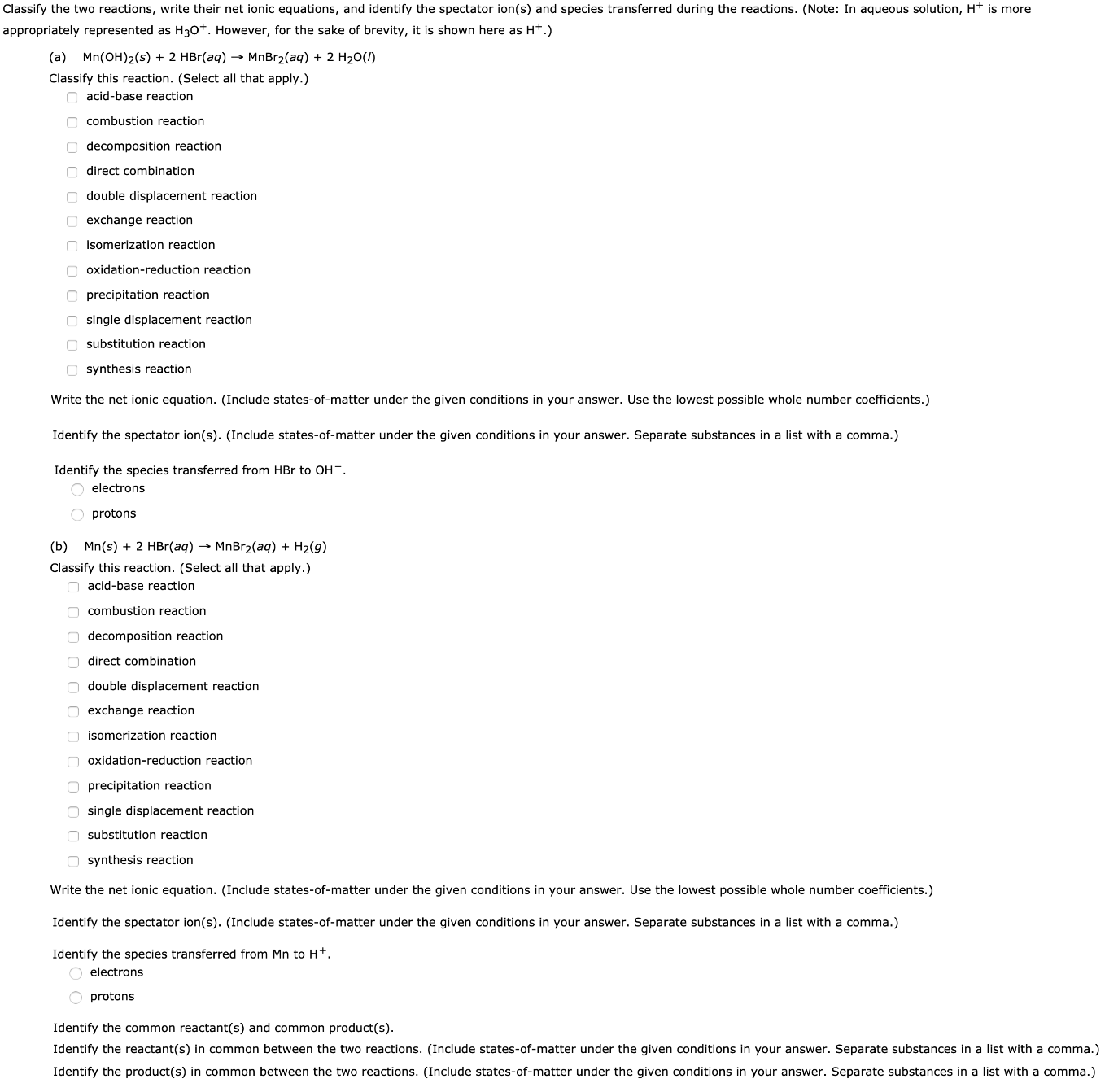# Classify the two reaction, write their net ionic equations, and identify the spectator ion(s) and species transferred during the reactions. (Note: In aqueous solution, H+ is more appropriately represented as H3O+. However, for the sake of brevity, it is shown here as H+.) (a) Mn(OH)2(s) + 2 HBr(aq) -> MnBr2(aq) + 2 H2O(l) Classify this reaction. Write the net ionic equation. Identify the spectator ion(s). Identify the species transferred from HBr to OH-. (b) Mn(s) + 2 HBr(aq) -> MnBr2(aq) + H2(g) Classify this reaction. Write the net ionic equation. Identify the spectator ion(s). Identify the species transferred from Mn to H+. Identify the common reactant(s) and common product(s). Identify the reactant(s) in common between the two reactions. (Include states-of-matter under the given conditions in your answer. Separate substances in a list with a comma.) Identify the product(s) in common between the two reactions. (Include states-of-matter under the given conditions in your answer. Separate substances in a list with a comma.)